# Physics Test Flashcards

Set Details Share
created 10 years ago by XxIraxX
10,177 views
Page to share:
Embed this setcancel
COPY
code changes based on your size selection
Size:
X

1

What is the wavelength of microwaves of 3.0 ´ 109 Hz frequency?
a.
0.060 m
c.
0.050 m
b.
0.10 m
d.
0.20 m

Use v = fl with v = 3 x 10^8 m/s

B

2

What is the frequency of infrared light of 1.0 ´ 10–4 wavelength?
a.
3.0 ´ 10–2 Hz
c.
3.0 ´ 1012 Hz
b.
3.0 ´ 104 Hz

Use v = fl with v = 3 x 10^8 m/s

C

3

In a vacuum, electromagnetic radiation of short wavelengths
a.
travels as fast as radiation of long wavelengths.
b.
travels slower than radiation of long wavelengths.
c.
travels faster than radiation of long wavelengths.
d.
can travel both faster and slower than radiation of long wavelengths.

Speed of light in a vacuum is constant, regardless of wavelengths

A

4

If you know the wavelength of any form of electromagnetic radiation, you can determine its frequency because
a.
all wavelengths travel at the same speed.
b.
the speed of light varies for each form.
c.
wavelength and frequency are equal.
d.
the speed of light increases as wavelength increases.

v = fl

A

5

5.

A highly polished finish on a new car provides a ____ surface for ____ reflection.
a.
rough; diffused
c.
rough; regular
b.
specular; diffused
d.
smooth; specular

The polished finish provides a smooth surface that produces a clear image, which is a specular reflection.

D

6

When a straight line is drawn perpendicular to a flat mirror at the point where an incoming ray strikes the mirror’s surface, the angles of incidence and reflection are measured from the normal and
a.
the angles of incidence and reflection are equal.
b.
the angle of incidence is greater than the angle of reflection.
c.
the angle of incidence is less than the angle of reflection.
d.
the angle of incidence can be greater than or less than the angle of reflection.

Law of reflection states the angles are equal

A

7

If a light ray strikes a flat mirror at an angle of 27° from the normal, the reflected ray will be
a.
27° from the mirror’s surface.
c.
90° from the mirror’s surface.
b.
27° from the normal.
d.
63° from the normal.

Law of reflection states angles are measured from the normal

B

8

8.

The image of an object in a flat mirror is always
a.
larger than the object.
c.
independent of the size of the object.
b.
smaller than the object.
d.
the same size as the object.

Plane mirrors produce images that are the same size

D

9

If you stand 3.0 m in front of a flat mirror, how far away from you would your image be in the mirror?
a.
1.5 m
c.
6.0 m
b.
3.0 m
d.
12.0 m

Image distance equals object distance for a plane mirror

C

10

Which of the following best describes the image produced by a flat mirror?
a.
virtual, inverted, and magnification greater than one
b.
real, inverted, and magnification less than one
c.
virtual, upright, and magnification equal to one
d.
real, upright, and magnification equal to one

Same size means magnification is one, image behind the mirror is virtual and upright

C

11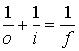A concave mirror with a focal length of 10.0 cm creates a real image 30.0 cm away on its principal axis. How far from the mirror is the corresponding object?
a.
20.0 cm
c.
7.50 cm
b.
15.0 cm
d.
5.00 cm

f = 10 and i = 30

D

12If a virtual image is formed 10.0 cm along the principal axis from a convex mirror with a focal length of –15.0 cm, what is the object’s distance from the mirror?
a.
30.0 cm
c.
6.0 cm
b.
12 cm

i = -10 and f = -15

A

13A convex mirror with a focal length of –20.0 cm forms an image 12 cm behind the surface. Where is the object as measured from the surface?
a.
7.5 cm
c.
22 cm
b.
15 cm
d.
3.0 ´ 101 cm

i = -12 and f = -20

D

14

When red light and green light shine on the same place on a piece of white paper, the spot appears to be
a.
yellow.
c.
white.
b.
brown.
d.
black.

Red and green combine to produce yellow light

A

15A concave mirror forms a real image at 25 cm from the mirror surface along the principal axis. If the corresponding object is at a 10.0 cm distance, what is the mirror’s focal length?
a.
1.4 cm
c.
12 cm
b.
17 cm
d.
7.1 cm

o = 10 and i = 25

D

16

Which of the following is NOT an additive primary color?
a.
yellow
c.
red
b.
blue
d.
green

The additive primary colors are red, green, and blue

A

17

What color does yellow pigment subtract from white light?
a.
blue
c.
yellow
b.
red
d.
green

Yellow subtracts its compliment, which is blue

A

18

Which of the following is NOT a primary subtractive color?
a.
yellow
c.
magenta
b.
cyan
d.
blue

Subtractive primary colors are yellow, cyan, and magenta

D

19

When the transmission axis is perpendicular to the plane of polarization for light,
a.
all the light passes through.
c.
little of the light passes through.
b.
most of the light passes through.
d.
no light passes through.

If the polarized light is perpendicular to the transmission axis, no light is let through

D

20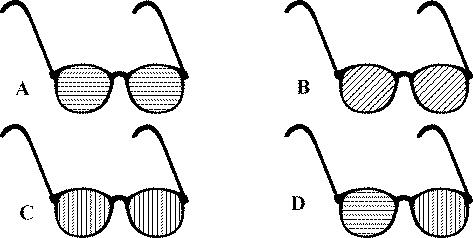Which pair of glasses is best suited for automobile drivers? The transmission axes are shown by straight lines on the lenses. (Hint: The light reflects off the hood of the car.)

Light reflected off a horizontal surface is horizontally polarized, so to be effective the glasses should have a vertical transmission axis

C

21

If you looked at a light through the lenses from two polarizing sunglasses that were overlapped at right angles to one another,
a.
all of the light would pass through.
c.
little of the light would pass through.
b.
most of the light would pass through.
d.
none of the light would pass through.

Perpendicular polarizers will allow no light to go through.

D

22

Part of a pencil that is placed in a glass of water appears bent in relation to the part of the pencil that extends out of the water. What is this phenomenon called?
a.
interference
c.
diffraction
b.
refraction
d.
reflection

Light passing from one material to another is bent due to refraction

B

23

When a light ray passes from water (n = 1.333) into diamond (n = 2.419) at an angle of 45°, its path is
a.
bent toward the normal.
c.
parallel to the normal.
b.
bent away from the normal.
d.
not bent.

When going from a lower index to a higher index the light is bent toward the normal

A

24

When a light ray passes from zircon (n = 1.923) into fluorite (n = 1.434) at an angle of 60°, its path is
a.
bent toward the normal.
c.
parallel to the normal.
b.
bent away from the normal.
d.
not bent.

When going from a high index to a lower index the light is bent away from the normal

B

25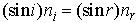A beam of light in air is incident at an angle of 35° to the surface of a rectangular block of clear plastic (n = 1.49). What is the angle of refraction?
a.
42°
c.
55°
b.
23°
d.
59°

Use Snell's Law , with i = 35, ni = 1.0, and nr = 1.49

B

26An object is placed along the principal axis of a thin converging lens that has a focal length of 30.0 cm. If the distance from the object to the lens is 40.0 cm, what is the distance from the image to the lens?
a.
17.3 cm
c.
1.20 m
b.
–17.3 cm
d.
–1.20 m

f = 30 and o = 40

C

27An object is placed 20.0 cm from a thin converging lens along the axis of the lens. If a real image forms behind the lens at a distance of 8.00 cm from the lens, what is the focal length of the lens?
a.
30.0 cm
c.
15.0 cm
b.
10.0 cm
d.
5.71 cm

o = 20 and i = 8

D

28An object is placed 14.0 cm from a diverging lens. If a virtual image appears 10.0 cm from the lens on the same side as the object, what is the focal length of the lens?
a.
–50.0 cm
c.
–10.0 cm
b.
–35 cm
d.
–8.0 cm

o = 14 and i = -10

B

29A candle that is 10.0 cm high is placed 30.0 cm in front of a diverging lens. The lens has a focal length of –20.0 cm. What is the magnification of the image?
a.
2.50
c.
0.400
b.
–0.400
d.
–2.50

o = 30 and f = -20 gives and image distance of -12 cm and M = 0.4C

30

Which of the following describes what will happen to a light ray incident on a glass-to-air boundary at greater than the critical angle?
a.
total reflection
c.
partial reflection, partial transmission
b.
total transmission
d.
partial reflection, total transmission

Greater than critical angle means total internal reflection

A

31

In a double-slit interference pattern the path length from one slit to the first dark fringe of a double-slit interference pattern is longer than the path length from the other slit to the fringe by
a.
three-quarters of a wavelength.
c.
one-quarter of a wavelength.
b.
one-half of a wavelength.
d.
one full wavelength.

Dark fringe means destructive interference so the path lengths have to be one-half wavelength different

B

32

Interference effects observed in the early 1800s were instrumental in supporting a concept of the existence of which property of light?
a.
polarization
c.
wave nature
b.
particle nature
d.
electromagnetic character

Only waves interfere

C

33

If light waves are coherent,
a.
they shift over time.
b.
their intensity is less than that of incoherent light.
c.
they remain in phase.
d.
they have less than three different wavelengths.

Coherent light is in phase

C

34

Which of the following is the time it takes to complete a cycle of motion?
a.
amplitude
c.
frequency
b.
period
d.
revolution

B

35

Which of the following is the number of cycles or vibrations per unit of time?
a.
amplitude
c.
frequency
b.
period
d.
revolution

C

36

How are frequency and period related?
a.
They are directly related.
b.
They are inversely related.
c.
They both measure the time per cycle.
d.
They both measure the number of cycles per unit of time.

B

37

An amusement park ride has a frequency of 0.05 Hz. What is the ride’s period?
a.
5 s
c.
20 s
b.
10 s
d.
40 s

C

38

Which of the following is a single nonperiodic disturbance?
a.
pulse wave
c.
sine wave
b.
periodic wave
d.
transverse wave

A

39

Which of the following is a wave whose source is some form of repeating motion?
a.
pulse wave
c.
sine wave
b.
periodic wave
d.
transverse wave

B

40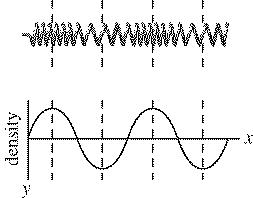In the waveform of the longitudinal wave shown above, the compressed regions correspond to
a.
the wavelength.
c.
troughs.
b.
crests.
d.
the mass

B

41

In the waveform of the longitudinal wave shown above, the stretched regions correspond to
a.
the wavelength.
c.
troughs.
b.
crests.
d.
the mass.

C

42

A periodic wave has a wavelength of 0.50 m and a speed of 20 m/s. What is the wave frequency?
a.
0.02 Hz
c.
40 Hz
b.
20 Hz
d.
10 Hz

C

43

A musical tone sounded on a piano has a frequency of 410 Hz and a wavelength of 0.80 m. What is the speed of the sound wave?
a.
170 m/s
c.
330 m/s
b.
240 m/s
d.
590 m/s

C

44

Two waves can occupy the same space at the same time because waves
a.
are matter.
c.
do not cause interference patterns.
b.
are displacements of matter.
d.
cannot pass through one another.

B

45

Standing waves are produced by periodic waves of
a.
any amplitude and wavelength traveling in the same direction.
b.
the same amplitude and wavelength traveling in the same direction.
c.
any amplitude and wavelength traveling in opposite directions.
d.
the same frequency, amplitude, and wavelength traveling in opposite directions.

D

46

Which of the following wave lengths would produce standing waves on a string approximately 3.5 m long?
a.
2.33 m
c.
3.75 m
b.
2.85 m
d.
4.55 m

A

47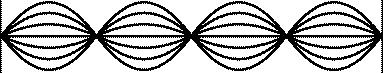How many nodes and antinodes are shown in the standing wave above?
a.
four nodes and four antinodes
c.
four nodes and five antinodes
b.
four nodes and three antinodes
d.
five nodes and four antinodes

D

48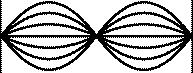How many nodes and antinodes are shown in the standing wave above?
a.
two nodes and three antinodes
c.
one-third node and one antinode
b.
one node and two antinodes
d.
three nodes and two antinodes

D

49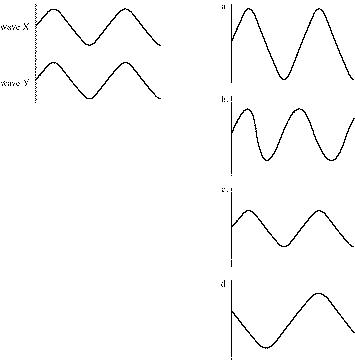In the diagram above, use the superposition principle to find the resultant wave of waves X and Y.
a.
a
c.
c
b.
b
d.
d

A

50In the diagram above, use the superposition principle to find the resultant wave of waves Q and R.
a.
a
c.
c
b.
b
d.
d

B

51

Sound waves
a.
are a part of the electromagnetic spectrum.
b.
do not require a medium for transmission.
c.
are longitudinal waves.
d.
are transverse waves.

C

52

Which of the following is the region of a longitudinal wave in which the density and pressure are greater than normal?
a.
rarefaction
c.
spherical wave
b.
compression
d.
Doppler effect

B

53

Which of the following is the region of a longitudinal wave in which the density and pressure are less than normal?
a.
rarefaction
c.
spherical wave
b.
compression
d.
Doppler effect

A

54

Pitch refers to
a.
how many vibrations per second are perceived by the human ear.
b.
how many cycles per second are in a transverse wave.
c.
the constructive interference of electromagnetic waves.
d.
the destructive interference in transverse waves.

A

55

Which has a higher speed of sound?
a.
water
c.
methyl alcohol
b.
oxygen
d.
copper

D

56

Which statement about sound waves is correct?
a.
They generally travel faster through solids than through gases.
b.
They generally travel faster through gases than through solids.
c.
They generally travel faster through gases than liquids.
d.
They generally travel faster than light.

A

57

As a sound source approaches and then moves past a stationary observer, the observer will hear
a.
c.
a rise in pitch, then a drop in pitch.
b.
a sudden drop in pitch.
d.
a drop in pitch, then a rise in pitch.

C

58

If you hear the pitch of a siren become lower, you know that
a.
neither you nor the siren is moving.
b.
you are moving toward the siren or the siren is moving toward you.
c.
you are moving away from the siren or the siren is moving toward you.
d.
the source has just passed you or it is accelerating away from you.

D

59

If you are on a train, how will the pitch of the train’s whistle sound to you as the train moves?
a.
The pitch will become steadily higher.
b.
The pitch will become steadily lower.
c.
The pitch will not change.
d.
The pitch will become higher then become lower.

C

60

Tripling the distance from a sound source will change the intensity of the sound waves by what factor?

1/9

61

What is the intensity of sound waves produced by a trumpet at a distance of 1.6 m when the power output of the trumpet is 0.30 W?
a.
5.9 ´ 10–3 W/m2
c.
9.4 ´ 10–3 W/m2
b.
1.5 ´ 10–2 W/m2
d.
3.7 ´ 10–2 W/m2

C

62

If the intensity of a sound is increased by a factor of 100, the new decibel level will be
a.
two units greater.
c.
10 times greater.
b.
double the old one.
d.
20 units greater.

D

63

Which of the following is the condition that exists when the frequency of a force applied to a system matches the natural frequency of vibration of the system?
a.
pitch
c.
timbre
b.
decibel level
d.
resonance

D

64

A sound twice the intensity of the faintest audible sound is not perceived as twice as loud because the sensation of loudness is
a.
approximately logarithmic in the human ear.
b.
approximately exponential in the human ear.
c.
outside the threshold of hearing.
d.
outside the threshold of pain.

A

65

If both ends of a pipe are open,
a.
all harmonics are present.
c.
only odd harmonics are present.
b.
no harmonics are present.
d.
only even harmonics are present.

A

66

If one end of a pipe is closed,
a.
all harmonics are present.
c.
only odd harmonics are present.
b.
no harmonics are present.
d.
only even harmonics are present.

C

67

What is the lowest frequency that will resonate in a 2.0 m length organ pipe closed at one end? The speed of sound in air at this temperature is 340 m/s.
a.
42 Hz
c.
170 Hz
b.
85 Hz
d.
680 Hz

A

68

If a guitar string has a fundamental frequency of 500 Hz, what is the frequency of its second harmonic?
a.
250 Hz
c.
1000 Hz
b.
750 Hz
d.
1500 Hz

C

69

The effects of sound on the ear are loudness, pitch, and quality. Loudness is an effect of ____, pitch is an effect of ____, and timbre is an effect of ____.
a.
intensity; harmonic content; frequency
c.
frequency; intensity; harmonic content
b.
harmonic content; frequency; intensity
d.
intensity; frequency; harmonic content

D

70

What phenomenon is created by two tuning forks side by side that emit frequencies that differ by only a small amount?
a.
resonance
c.
the Doppler effect
b.
interference
d.
beats

D

71

Two vibrating tuning forks held side by side will create a beat frequency of what value if the individual frequencies of the two forks are 342 Hz and 345 Hz, respectively?
a.
687 Hz
c.
339 Hz
b.
343.5 Hz
d.
3 Hz

D

72

Two notes have a beat frequency of 4 Hz. The frequency of one note is 420 Hz. What is the frequency of the other note?
a.
422 Hz or 418 Hz
c.
424 Hz or 416 Hz
b.
105 Hz
d.
1680 Hz

C

73

Beats are formed by the interference of two waves
a.
of slightly different frequencies traveling in the same direction.
b.
of slightly different frequencies traveling in different directions.
c.
with equal frequencies traveling in the same direction.
d.
with equal frequencies traveling in different directions.

A

74

What happens when a rubber rod is rubbed with a piece of fur, giving it a negative charge?
a.
Protons are removed from the rod.
c.
The fur is also negatively charged.
b.
Electrons are added to the rod.
d.
The fur is left neutral.

B

75

A repelling force occurs between two charged objects when
a.
charges are of unlike signs.
c.
charges are of equal magnitude.
b.
charges are of like signs.
d.
charges are of unequal magnitude.

B

76

An attracting force occurs between two charged objects when
a.
charges are of unlike signs.
c.
charges are of equal magnitude.
b.
charges are of like signs.
d.
charges are of unequal magnitude.

A

77

Electric charge is
a.
found only in a conductor.
c.
found only in insulators.
b.
conserved.
d.
not conserved.

B

78

Which sentence best characterizes electric conductors?
a.
They have low mass density.
b.
They have high tensile strength
c.
They have electric charges that move freely.
d.
They are poor heat conductors.

C

79

Which sentence best characterizes electric insulators?
a.
Charges on their surface do not move.
c.
Electric charges move freely in them.
b.
They have high tensile strength
d.
They are good heat conductors.

A

80

The process of charging a conductor by bringing it near another charged object and then grounding the conductor is called
a.
charging by contact.
c.
charging by polarization
b.
induction.
d.
neutralization.

B

81

Both insulators and conductors can be charged by
a.
grounding.
c.
polarization.
b.
induction
d.
contact.

D

82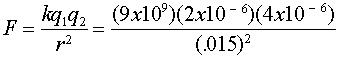If two point charges are separated by 1.5 cm and have charge values of 2.0 mC and –4.0 mC, respectively, what is the value of the mutual force between them? (kc = 8.99 ´ 109 N·m2/C2)
a.
320 N
c.
8.0 ´ 10–12
b.
3.6 ´ 10–8 N
d.
3.1 ´ 10–3 N

Use Coulomb's Law

A

83Consider a thundercloud that has an electric charge of 40.0 C near the top of the cloud and –40.0 C near the bottom of the cloud. These charges are separated by about 2.0 km. What is the magnitude of the electric force between these two sets of charges? (kc = 8.99 ´ 109 N·m2/C2)
a.
3.6 ´ 104 N
c.
3.6 ´ 106 N
b.
3.6 ´ 105 N
d.
3.6 ´ 107 N

C

84

If the charge and mass are tripled for two identical charges maintained at a constant separation, the electric and gravitational forces between them will be changed by what factor?
a.
9
c.
1/9
b.
2/3
d.
18

A

85

Two point charges, initially 1 cm apart, are moved to a distance of 3 cm apart. By what factor do the resulting electric and gravitational forces between them change?
a.
3
c.
1/3
b.
9
d.
1/9

D

86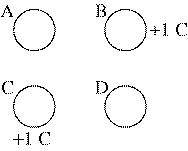Four charges—A, B, C, and D— are at the corners of a square. Charges A and D, on opposite corners, have equal charge, whereas both B and C have a charge of 1.0 C. If the force on B is zero, what is the charge on A?
a.
–1.0 C
c.
–0.35 C
b.
–0.20 C
d.
–0.71 C

C

87

Charge A and charge B are 2 m apart. Charge A is 1 C and charge B is 2 C. Charge C, which is 2 C, is located between them, and the force on charge C is zero. How far from charge A is charge C?
a.
1 m
c.
0.8 m
b.
0.7 m
d.
0.5 m

C

88

Two equal positive charges, both q = 2.0 ´ 10–6 C, interact with a third charge, Q = 4.0 ´ 10–6 C, as shown in the figure above. What is the magnitude of the electric force on Q?
a.
0.23 N
c.
0.46 N
b.
–0.17 N
d.
0.60 N

C

89

Two point charges have a value of 30 mC each and are 4 cm apart. What is the electric field at the midpoint between the two charges? (kc = 8.99 ´ 109 N·m2/C2)
a.
4.5 ´ 107 N/C
c.
.5 ´ 107 N/C
b.
2.3 ´ 107 N/C
d.
0 N/C

D

90

At what point is the electric field of an isolated, uniformly charged, hollow metallic sphere greatest?
a.
at the center of the sphere
c.
at infinity
b.
at the sphere’s inner surface
d.
at the sphere’s outer surface

D

91

If a conductor is in electrostatic equilibrium, any excess charge
a.
flows to the ground.
b.
resides entirely on the conductor’s outer surface.
c.
resides entirely on the conductor’s interior.
d.
resides entirely in the center of the conductor.

B

92

If an irregularly-shaped conductor is in electrostatic equilibrium, charge accumulates
a.
where the radius of curvature is smallest.
c.
evenly throughout the conductor.
b.
where the radius of curvature is largest.
d.
in flat places.

A

93

A proton (q = 1.60 ´ 10 - 19 C) moves 10.0 cm on a path parallel to the direction of a uniform electric field of strength 3.0 N/C. What is the change in electrical potential energy?
a.
4.8 ´ 10–20 J
c.
–4.8 ´ 10–20 J
b.
1.6 ´ 10–20 J
d.
–1.6 ´ l0–20 J

C

94

When an electron (e = –1.6 ´ 10–19 C) moves 0.10 m along the direction of an electric field with a strength of 3.0 N/C, what is the magnitude of the potential difference between the electron’s initial and final points?
a.
4.8 ´ 10–19 V
c.
0.03 V
b.
0.30 V
d.
3.0 ´ 101 V

B

95

A uniform electric field with a magnitude of 500 N/C is directed parallel to the positive x-axis. If the potential at x = 5 m is 2500 V, what is the potential at x = 2 m?
a.
1000 V
c.
4000 V
b.
2000 V
d.
4500 V

C

96

Charge build up between the plates of a capacitor stops when
a.
there is no net charge on the plates.
b.
unequal amounts of charge accumulate on the plate.
c.
the potential difference between the plates is equal to the potential difference between the terminals of the battery.
d.
the charge on both plates is the same.

C

97

When comparing the net charge of a charged capacitor with the net charge of the same capacitor when it is uncharged, the net charge is
a.
greater in the charged capacitor.
b.
less in the charged capacitor.
c.
equal in both capacitors.
d.
greater or less in the charged capacitor, but never equal.

C

98

When a capacitor discharges,
a.
it must be attached to a battery.
b.
charges move back from one plate to another through the circuit until both plates are uncharged.
c.
charges move from one plate to another until equal and opposite charges accumulate on the plates.
d.
it cannot be connected to a material that conducts.

B

99

A capacitor consists of two metal plates; ____ is stored on one plate and ____ is stored on the other.
a.
negative charge; positive charge
c.
potential difference; internal resistance
b.
potential energy; kinetic energy
d.
residual charge; induced charge

A

100

Increasing the potential difference across the two plates of a capacitor will produce what effect on the capacitor?
a.
It will increase the charge.
c.
It will increase the capacitance.
b.
It will decrease the charge.
d.
It will decrease the capacitance.

A

101

A 0.25 mF capacitor is connected to a 9.0 V battery. What is the charge on the capacitor?
a.
1.2 ´ 10–12 C
c.
2.8 ´ 10–8 C
b.
2.2 ´ 10–6 C
d.
3.6 ´ 10–7 C

B

102

A 0.50 mF capacitor is connected to a 12 V battery. How much electrical potential energy is stored in the capacitor?
a.
2.0 ´ 10–12 J
c.
0.04 J
b.
1.0 ´ 10–12 J
d.
3.6 ´ 10–5 J